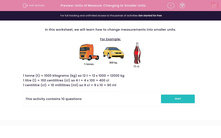# Units of Measure: Changing to Smaller Units

This worksheet tests the conversion of measurements into smaller unitsThis content is premium and exclusive to EdPlace subscribers.Key stage:  KS 2

Curriculum topic:   Maths and Numerical Reasoning

Curriculum subtopic:   Metric & Imperial

Difficulty level:#### Worksheet Overview

In this worksheet, we will learn how to change measurements into smaller units.

For Example:1 tonne (t) = 1000 kilograms (kg) so 12 t = 12 x 1000 = 12000 kg

1 litre (l) = 100 centilitres (cl) so 4 l = 4 x 100 = 400 cl

1 centilitre (cl) = 10 millilitres (ml) so 9 cl = 9 x 10 = 90 ml

### What is EdPlace?

We're your National Curriculum aligned online education content provider helping each child succeed in English, maths and science from year 1 to GCSE. With an EdPlace account you’ll be able to track and measure progress, helping each child achieve their best. We build confidence and attainment by personalising each child’s learning at a level that suits them.

Get started